# Standard Form

GCSEKS3Level 4-5AQACambridge iGCSEEdexcelEdexcel iGCSEOCRWJEC

## Standard Form

Standard form is a shorthand way of expressing VERY LARGE or VERY SMALL numbers. There are 6 key skills that you need to learn.

Standard from to ordinary numbers and ordinary numbers to standard form are two skills you will be taught.

Make sure you are happy with the following topics before continuing:

Level 4-5GCSEKS3AQAEdexcelOCRWJECCambridge iGCSEEdexcel iGCSE

## What is Standard Form?

We always write a number in standard form exactly like this:

$\textcolor{red}{A}\times\textcolor{blue}{10}^\textcolor{limegreen}{n}$

The Three Key Rules

1)   $\textcolor{red}{A}$ must be any between $\mathbf{1}$ and $\mathbf{10}$, in other words, $1\leq A<10$.

2)   Standard form is always $\textcolor{blue}{10}$ to the power of something ($\textcolor{limegreen}{n}$)

3)   $\textcolor{limegreen}{n}$ must be a whole number, this is the number of places the decimal point moves.

e.g. $\textcolor{red}{4.2}\times\textcolor{blue}{10}^\textcolor{limegreen}{5}$

Level 4-5GCSEKS3AQAEdexcelOCRWJECCambridge iGCSEEdexcel iGCSE

## Skill 1: Standard Form into Large Numbers

Example: Express $4.2\times\textcolor{limegreen}{10^5}$ as a number not in standard form.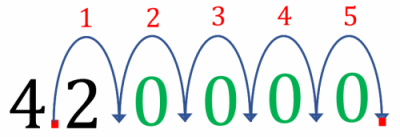Firstly, recall that $\textcolor{limegreen}{10^5}=\textcolor{limegreen}{10}\times\textcolor{limegreen}{10}\times\textcolor{limegreen}{10}\times\textcolor{limegreen}{10}\times\textcolor{limegreen}{10}$.

Then, we get  $4.2\times10^5=4.2\times10\times10\times10\times10\times10$

Multiplying by $10$ means moving the decimal place to the right, here we must do it $5$ times:

Step 1: Write $4\textcolor{red}{.}2$ out and move the decimal place $\textcolor{red}{5}$ jumps to the right.

Step 2: Add $\textcolor{limegreen}{\mathbf{0}}$‘s to fill in the spaces created as the decimal point has moved.

Step 3: Remove the original decimal point.

This gives the answer to be:

$4.2\times10^{5}=420000$

Level 4-5GCSEKS3AQAEdexcelOCRWJECCambridge iGCSEEdexcel iGCSE

## Skill 2: Standard Form into Small Numbers

Example: Write $2.8\times\textcolor{limegreen}{10^{-4}}$ in decimal notation.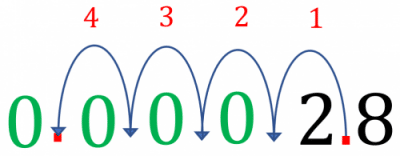This is different because the power is negative, but it’s actually no harder. We know,

$\textcolor{limegreen}{10^{-4}}=\textcolor{limegreen}{\dfrac{1}{10^4}}$

This means we are $\textcolor{limegreen}{\text{dividing by }10}$ $\textcolor{limegreen}{\text{four times}}$ which means we move the decimal point $4$ spaces to the left.

Step 1: Write $2\textcolor{red}{.}8$ out and move the decimal place $\textcolor{red}{4}$ jumps to the left.

Step 2: Add $\textcolor{limegreen}{\mathbf{0}}$‘s to fill in the space created as the decimal point has moved.

Step 3: Remove the original decimal point.

Therefore, we have concluded that

$2.8\times10^{-4}= 0.00028$

Level 4-5GCSEKS3AQAEdexcelOCRWJECCambridge iGCSEEdexcel iGCSE
Level 4-5GCSEKS3AQAEdexcelOCRWJECCambridge iGCSEEdexcel iGCSE

## Skill 3: Writing Large Numbers in Standard Form

Example: Write $56,700,000$ in standard form.

Step 1: Move the decimal point to the left until the number becomes $5.67$ $(1\leq A<10)$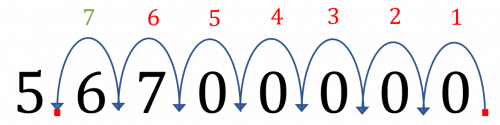Step 2: Count the number of times the decimal point has moved to the left, this will become our power ($\textcolor{limegreen}{n}$), in this case $\textcolor{limegreen}{7}$.

Step 3: We have moved to the left meaning it will be $\textcolor{limegreen}{+7}$ not $-7$

So,

$56,700,000 = 5.67\times10^{\textcolor{limegreen}{7}}$

Level 4-5GCSEKS3AQAEdexcelOCRWJECCambridge iGCSEEdexcel iGCSE

## Skill 4: Writing Small Numbers in Standard Form

Example: Write $0.0000099$ in standard form.

Step 1: Move the decimal point to the right until the number becomes $9.9$ $(1\leq A<10)$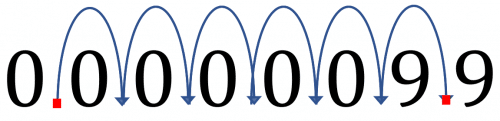Level 4-5GCSEKS3AQAEdexcelOCRWJECCambridge iGCSEEdexcel iGCSE
Level 4-5GCSEKS3AQAEdexcelOCRWJECCambridge iGCSEEdexcel iGCSE

## Skill 5: Multiplying Standard Form

Example: Find the standard form value of $(3\times10^8)\times(7\times10^4)$, without using a calculator.

Step 1: Change the order around of the things being multiplied.

$(\textcolor{red}{3}\times\textcolor{limegreen}{10^8})\times(\textcolor{red}{7}\times\textcolor{limegreen}{10^4})=\textcolor{red}{3}\times\textcolor{red}{7}\times\textcolor{limegreen}{10^8}\times\textcolor{limegreen}{10^4}$

Step 2: Multiply the numbers and the powers out separately.

$(\textcolor{red}{3}\times\textcolor{red}{7})\times(\textcolor{limegreen}{10^8}\times\textcolor{limegreen}{10^4})=\textcolor{red}{21}\times\textcolor{limegreen}{10^{12}}$

Step 3: Convert the number at the front to standard form if necessary ($1 \leq A < 10$).

This answer is not in standard form ($21$ is not between $1$ and $10$), and we need it to be. Fortunately, if we recognise that $\textcolor{red}{21}=\textcolor{red}{2.1}\textcolor{limegreen}{\times10}$, then we get that

$\textcolor{red}{21}\textcolor{limegreen}{\times10^{12}}=\textcolor{red}{2.1}\textcolor{limegreen}{\times10}\textcolor{limegreen}{\times10^{12}}=\textcolor{red}{2.1}\textcolor{limegreen}{\times10^{13}}$

Level 4-5GCSEKS3AQAEdexcelOCRWJECCambridge iGCSEEdexcel iGCSE

## Skill 6: Dividing Standard Form

Example: Find the standard form value of $(\textcolor{red}{8}\times\textcolor{limegreen}{10^{-5}})\div(\textcolor{red}{2}\times\textcolor{limegreen}{10^6})$, without using a calculator.

Step 1: Break up the problem and change the order of how we divide things.

$\dfrac{\textcolor{red}{8}\times\textcolor{limegreen}{10^{-5}}}{\textcolor{red}{2}\times\textcolor{limegreen}{10^6}}=\dfrac{\textcolor{red}{8}}{\textcolor{red}{2}}\times\dfrac{\textcolor{limegreen}{10^{-5}}}{\textcolor{limegreen}{10^6}}=\textcolor{red}{4}\times\textcolor{limegreen}{10^{-11}}$

Step 2: Convert the number at the front to standard form if necessary ($1 \leq A < 10$).

$\textcolor{red}{4}$ is between $1$ and $10$, so this answer is in standard form, and so we are done.

(Remember: $10^{-5} - 10^6 = 10^{-5-6} = 10^{-11}$)

Level 4-5GCSEKS3AQAEdexcelOCRWJECCambridge iGCSEEdexcel iGCSE

## Standard Form Example Questions

The power is negative, so this is going to be a very small number. As the power of ten is $-6$, we want to divide the number $1.15$ by $10$ six times, and so we will move the decimal point six places to the left.

$1.15\times10^{-6}=0.00000115$.Gold Standard Education

In this case, the power of $10$ is going to be positive.

So, if we move the decimal point in $5,980,000$ to the left six places it becomes $5.98$. Therefore, we get that,

$5,980,000=5.98\times10^{6}$Gold Standard Education

By considering the position where the first non-zero digit is compared to the units column we find,

$0.0068=6.8\times10^{-3}$

as the $6$ is $3$ places away from the units column.Gold Standard Education

First, write each of the numbers in standard form i.e.  $5.6\times10^6$ and $8\times10^2$

$(5.6\times10^6)\div(8\times10^2)=(5.6\div8)\times(10^6\div10^2)$

Using the formula $10^a\div10^b=10^{a-b}$ we can rewrite the eqaution as,

$(5.6\div8)\times10^{6-2}=0.7\times10^4$

Standard form requires the number be between $1$ and $10$, so adjusting by a factor of $10$, we have,

$0.7\times10^4=7×10^{-1} \times10^4=7\times10^3$Gold Standard Education

We will split up this multiplication, multiplying the initial numbers together and the powers of $10$ together separately. Firstly,

$2.5\times6=15$.

Secondly, using the multiplication law of indices,

$10^4\times10^{-9}=10^{4+(-9)}=10^{-5}$

So, we get

$(2.5\times10^{4})\times(6\times10^{-9}) =2.5\times6\times10^4\times10^{-9}\\ =15\times10^{-5}$

Standard form requires the number be between $1$ and $10$, so adjusting by a factor of $10$, we have,

$15\times10^{-5}=1.5\times10\times10^{-5}=1.5\times10^{-4}$Gold Standard Education

## Standard Form Worksheet and Example Questions

### (NEW) Standard Form - Exam Style Questions - MME

Level 4-5GCSEKS3NewOfficial MME

## Standard Form Drill Questions

Level 4-5GCSEKS3

### Standard Form (2) - Drill Worksheet

Level 4-5GCSEKS3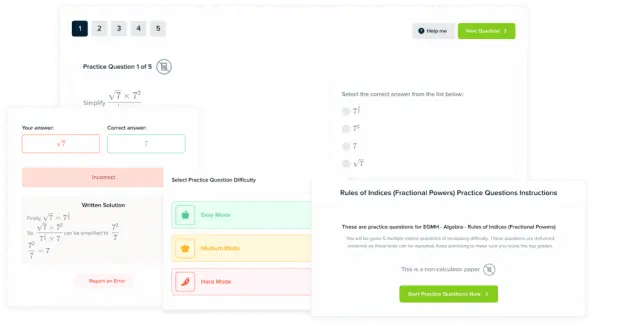Product

### £19.99

/month

Learn an entire GCSE course for maths, English and science on the most comprehensive online learning platform. With revision explainer videos & notes, practice questions, topic tests and full mock exams for each topic on every course, it’s easy to Learn and Revise with the MME Learning Portal.

Level 1-3GCSEKS3

Level 1-3GCSEKS3

Level 6-7